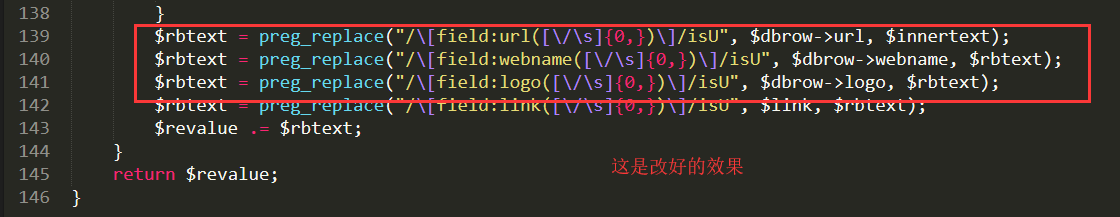# 织梦友情链接标签自定义输出bug修复

``````{dede:flink type='text' row='100'}
<a href="[field:url/]" target="_blank" rel="nofollow">[field:webname/]</a>
• 1
• 2
• 3

## 解决方法

``````\$rbtext = preg_replace("/[field:url([/s]{0,})]/isU", \$row['url'], \$innertext);
\$rbtext = preg_replace("/[field:webname([/s]{0,})]/isU", \$row['webname'], \$rbtext);
\$rbtext = preg_replace("/[field:logo([/s]{0,})]/isU", \$row['logo'], \$rbtext);``````

``````\$rbtext = preg_replace("/[field:url([/s]{0,})]/isU", \$dbrow->url, \$innertext);
\$rbtext = preg_replace("/[field:webname([/s]{0,})]/isU", \$dbrow->webname, \$rbtext);
\$rbtext = preg_replace("/[field:logo([/s]{0,})]/isU", \$dbrow->logo, \$rbtext);``````